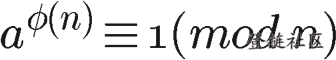## RSA算法原理

RSA算法的基于这样的数学事实：两个大质数相乘得到的大数难以被因式分解。 如：有很大质数p跟q，很容易算出N，使得 N = p * q， 但给出N, 比较难找p q（没有很好的方式， 只有不停的尝试）

1. 选取两个大质数p，q，计算N = p q 及 φ ( N ) = φ (p) φ (q) = (p-1) * (q-1)

三个数学概念： 质数(prime numbe)：又称素数，为在大于1的自然数中，除了1和它本身以外不再有其他因数。 互质关系：如果两个正整数，除了1以外，没有其他公因子，我们就称这两个数是互质关系（coprime）。 φ(N)：叫做欧拉函数，是指任意给定正整数N，在小于等于N的正整数之中，有多少个与N构成互质关系。

如果n是质数，则 φ(n)=n-1。 如果n可以分解成两个互质的整数之积， φ(n) = φ(p1p2) = φ(p1)φ(p2)。即积的欧拉函数等于各个因子的欧拉函数之积。

2. 选择一个大于1 小于φ(N)的数e，使得 e 和 φ(N)互质

e其实是1和φ(N)之前的一个质数

3. 计算d，使得de=1 mod φ(N) 等价于方程式 ed-1 = k φ(N) 求一组解。

d 称为e的模反元素，e 和 φ(N)互质就肯定存在d。

模反元素是指如果两个正整数a和n互质，那么一定可以找到整数b，使得ab被n除的余数是1，则b称为a的模反元素。 可根据欧拉定理证明模反元素存在，欧拉定理是指若n,a互质，则：a^φ(n) ≡ 1(mod n) 及 a^φ(n) = a * a^（φ(n) - 1）， 可得a的 φ(n)-1 次方，就是a的模反元素。

4. (N, e)封装成公钥，(N, d)封装成私钥。 假设m为明文，加密就是算出密文c: m^e mod N = c (明文m用公钥e加密并和随机数N取余得到密文c) 解密则是： c^d mod N = m　(密文c用密钥解密并和随机数N取余得到明文m)

私钥解密这个是可以证明的，这里不展开了。

## 加解密步骤

1. Alice 随机取大质数P1=53，P2=59，那N=53*59=3127，φ(N)=3016
2. 取一个e=3，计算出d=2011。
3. 只将N=3127，e=3 作为公钥传给Bob（公钥公开）
4. 假设Bob需要加密的明文m=89，c = 89^3 mod 3127=1394，于是Bob传回c=1394。 （公钥加密过程）
5. Alice使用c^d mod N = 1394^2011 mod 3127，就能得到明文m=89。 （私钥解密过程）

## 安全性分析

``````　　1. ed≡1 (mod φ(n))。只有知道e和φ(n)，才能算出d。
2. φ(n)=(p-1)(q-1)。只有知道p和q，才能算出φ(n)。
3. n=pq。只有将n因数分解，才能算出p和q。``````

## 补充模运算规则

1. 模运算加减法： (a + b) mod p = (a mod p + b mod p) mod p (a - b) mod p = (a mod p - b mod p) mod p
2. 模运算乘法： (a b) mod p = (a mod p b mod p) mod p
3. 模运算幂 a ^ b mod p = ((a mod p)^b) mod p

• 发表于 2017-11-15 10:53
• 阅读 ( 30905 )
• 学分 ( 20 )
• 分类：入门/理论